## What are the zeros of the quadratic function? y = x^2 – 2x – 15

Question

What are the zeros of the quadratic function?
y = x^2 – 2x – 15

in progress 0
3 months 2021-08-22T11:07:29+00:00 1 Answers 2 views 0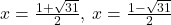Step-by-step explanation: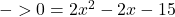-zeros means the solution aka x intercept, meaning y=0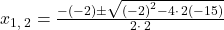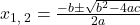where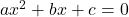=>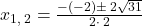simplify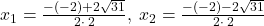Cannot simplify farther